International
Tables for
Crystallography
Volume D
Physical properties of crystals
Edited by A. Authier

International Tables for Crystallography (2006). Vol. D, ch. 1.8, pp. 226-227

## Section 1.8.5. Seebeck coefficient

G. D. Mahana*

aDepartment of Physics, 104 Davey Laboratory, Pennsylvania State University, University Park, Pennsylvania, USA
Correspondence e-mail: gmahan@psu.edu

### 1.8.5. Seebeck coefficient

| top | pdf |

The Seebeck coefficient S is the third transport coefficient that enters into the fundamental equations (1.8.2.1)and (1.8.2.2). Here we discuss some of its basic properties. First, we write down three integrals for the transport coefficients according to Goldsmid (1986):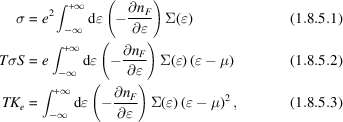whereis the chemical potential, e is the electron charge,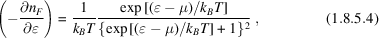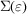, which we will call the transport distribution function, is given by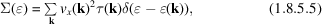where the summation is over the first Brillouin zone,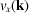is the group velocity of the carriers with wavevectorin the direction of the applied field,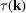is the lifetime of the carriers and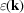is the dispersion relation for the carriers. In cases in which many bands contribute to the transport process, the summation has to be extended to all the bands. In some particular cases, such as for parabolic bands, the transport distribution defined in (1.8.5.5)takes a much simpler form: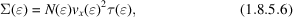where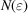is the density of states.

The Seebeck coefficient is defined in (1.8.5.2). Since the left-hand side of this equation contains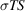, S is defined as the ratio of the two integrals in (1.8.5.1)and (1.8.5.2). The magnitude of the functionis immaterial for S, since the magnitude cancels in the ratio. All that matters is the dependence ofupon the energy. The function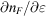is a symmetric function of. Furthermore, it becomes very small whenis more than a few thermal energies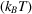away from the chemical potential. The Seebeck coefficient depends upon howvaries within this small energy range. The usual case is that it is a smooth function ofthat can be expanded in a Taylor series: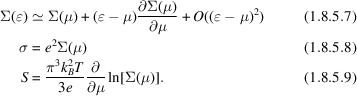The Seebeck coefficient has a linear dependence upon temperature. The coefficient of this term depends upon the energy variations inat the chemical potential. In most metals, a linear dependence upon temperature is observed (e.g. Rowe, 1995), particularly at high temperature. This linearity is found when one simple criterion is satisfied: that the functionhas a smooth dependence upon energy near the chemical potential.

Any deviation from linear behaviour in the Seebeck coefficient implies that the functionhas a more complicated behaviour near the chemical potential. Here we review several possible shapes. One is a simple Lorentzian peak: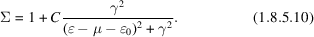The peak of the Lorentzian is atand the width is. Fig. 1.8.5.1shows the Seebeck coefficient calculated with this functional form. We arbitrarily took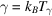and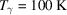. The four curves are for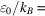10, 30, 100 and 300 K, and the largerhas the larger Seebeck coefficient. All of the curves have a broad peak as a function of temperature. If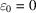, then, since the integrand is an odd function of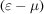. The vertical scale is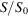where S0 = kB/e = 86.17 µV K−1. We see from Fig. 1.8.5.1that it is difficult to get values ofvery much larger than unity.Figure 1.8.5.1 | top | pdf |The Seebeck coefficient S in units of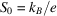forcontaining a Lorentzian peak. The values of S increase as the resonance energyincreases away from the chemical potential.

Another example is that of mixed-valence materials with f electrons. The f shells make electron states of narrow energy, which are approximated as Lorentzians. In this case,equals the inverse of the right-hand side of (1.8.5.10). The argument for this is that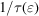is proportional to the right-hand side of (1.8.5.10). Since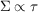, it contains the inverse of (1.8.5.10). Interestingly enough, plots of the Seebeck coefficient by Jaccard & Sierro (1982) for this case also contain broad peaks in energy, wherehas a maximum of about unity. In this case, a proper calculation includes the fact that both C andare functions of temperature. For a review, see Mahan (1997).

We give these examples of the Seebeck coefficient because they are the cases that occur most often. In many metals, the Seebeck coefficient is either linear with temperature or has broad peaks. The broad peaks are due to structure innear the chemical potential. This structure is usually due either to variations in the density of states or in the electron lifetime.

In insulators, the Seebeck coefficient can become relatively large. The exact value depends upon the energy gap, the temperature and the density of impurities. This example is treated in many references, e.g. Goldsmid (1986) and Rowe (1995).

### References

Goldsmid, H. J. (1986). Electronic refrigeration. London: Pion Limited.
Jaccard, D. & Sierro, J. (1982). Thermoelectric power of some intermediate valence compounds. In Valence instabilities, edited by P. Wachter & H. Boppart, pp. 409–413. Amsterdam: North-Holland.
Mahan, G. D. (1997). Good thermoelectrics. In Solid state physics, Vol. 51, edited by H. Ehrenreich & F. Spaepen, pp. 81–157. New York: Academic Press.
Rowe, D. M. (1995). Editor. CRC handbook of thermoelectrics. New York: CRC Press.